Скачать презентацию Quantum Information Stephen M Barnett University of Strathclyde

65415c816f6fdd497638f909d51b3980.ppt

• Количество слайдов: 40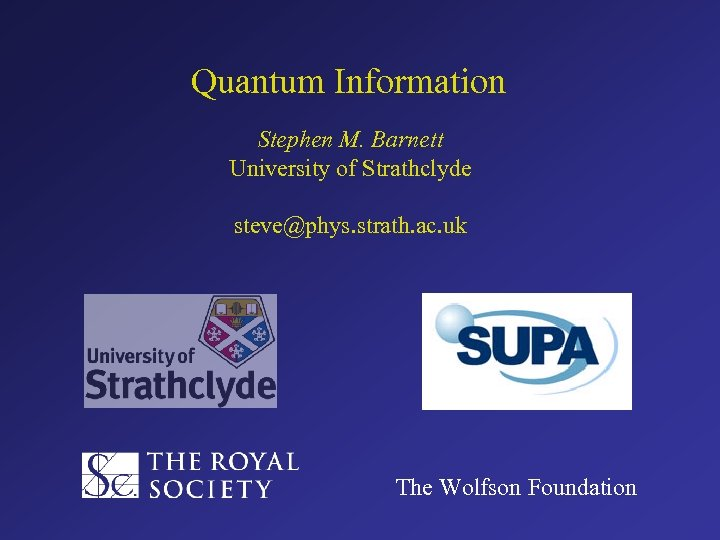Quantum Information Stephen M. Barnett University of Strathclyde steve@phys. strath. ac. uk The Wolfson Foundation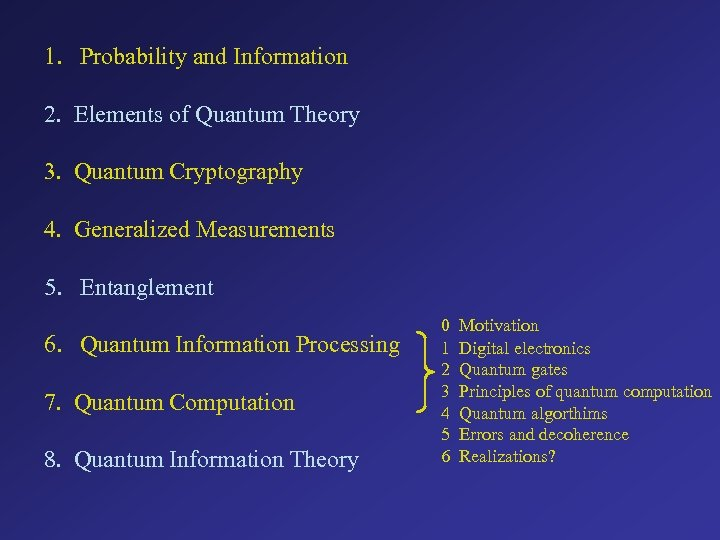1. Probability and Information 2. Elements of Quantum Theory 3. Quantum Cryptography 4. Generalized Measurements 5. Entanglement 6. Quantum Information Processing 7. Quantum Computation 8. Quantum Information Theory 0 1 2 3 4 5 6 Motivation Digital electronics Quantum gates Principles of quantum computation Quantum algorthims Errors and decoherence Realizations?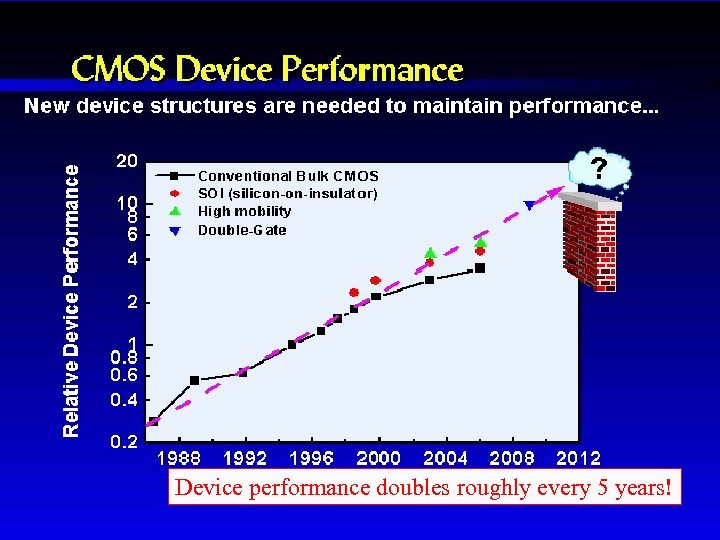CMOS Device Performance Device performance doubles roughly every 5 years!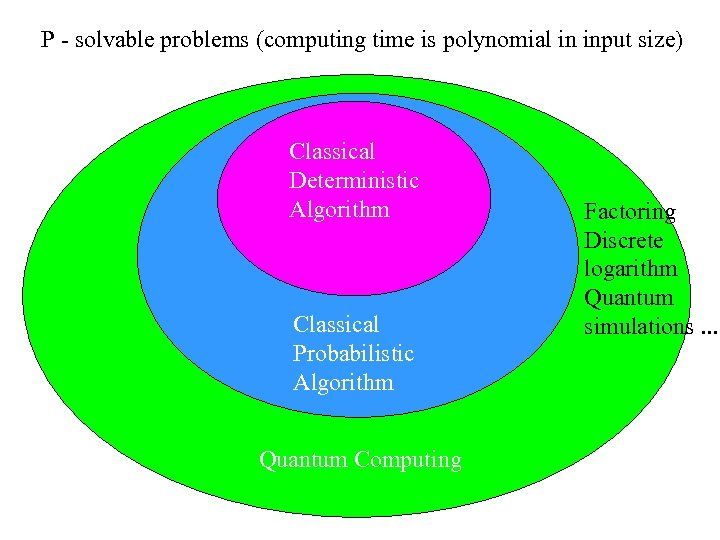P - solvable problems (computing time is polynomial in input size) Classical Deterministic Algorithm Classical Probabilistic Algorithm Quantum Computing Factoring Discrete logarithm Quantum simulations. . .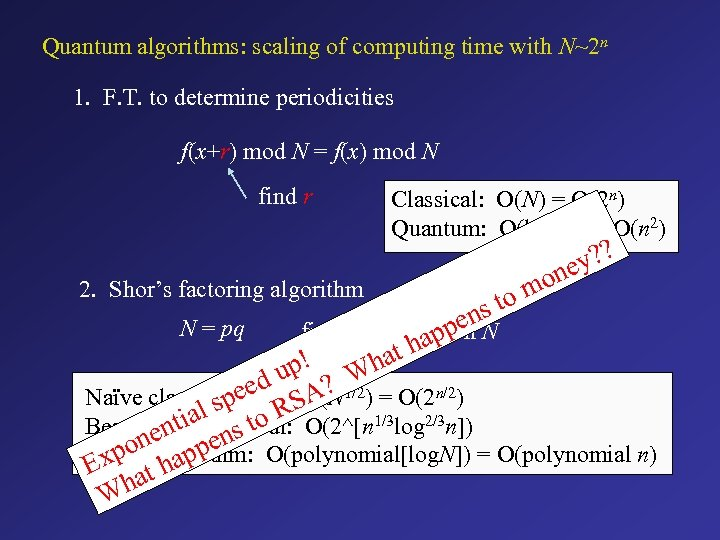Quantum algorithms: scaling of computing time with N~2 n 1. F. T. to determine periodicities f(x+r) mod N = f(x) mod N find r 2. Shor’s factoring algorithm Classical: O(N) = O(2 n) Quantum: O(log 2 N) = O(n 2) mo to y? ? ne s en. N N = pq find p and q given app at h up! Wh eed SO(N 1/2) = O(2 n/2) Naïve classicalp(trial): A? s ialclassical: O(2^[n 1/3 log 2/3 n]) t to R Best known nen pens O(polynomial[log. N]) = O(polynomial n) Shor’s algorithm: xpo hap E at Wh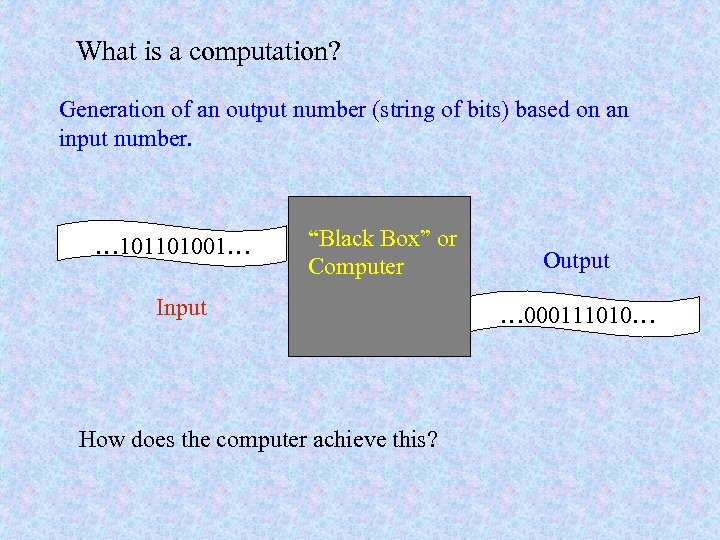What is a computation? Generation of an output number (string of bits) based on an input number. … 101101001… “Black Box” or Computer Input How does the computer achieve this? Output … 000111010…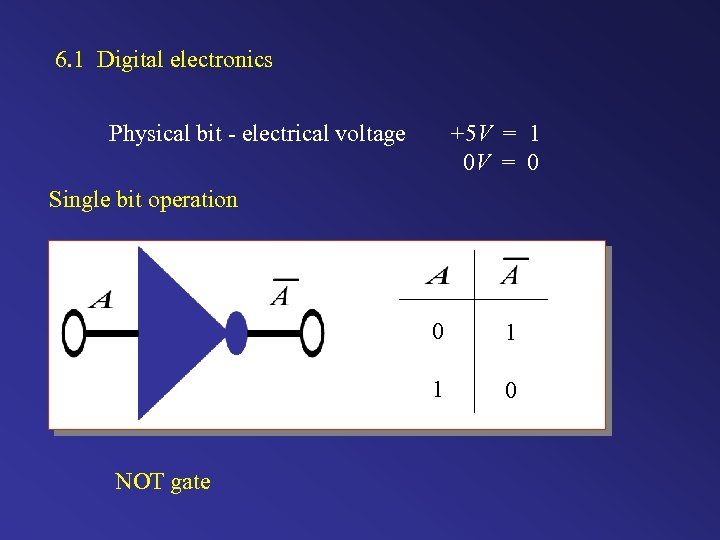6. 1 Digital electronics Physical bit - electrical voltage +5 V = 1 0 V = 0 Single bit operation 0 1 NOT gate 1 0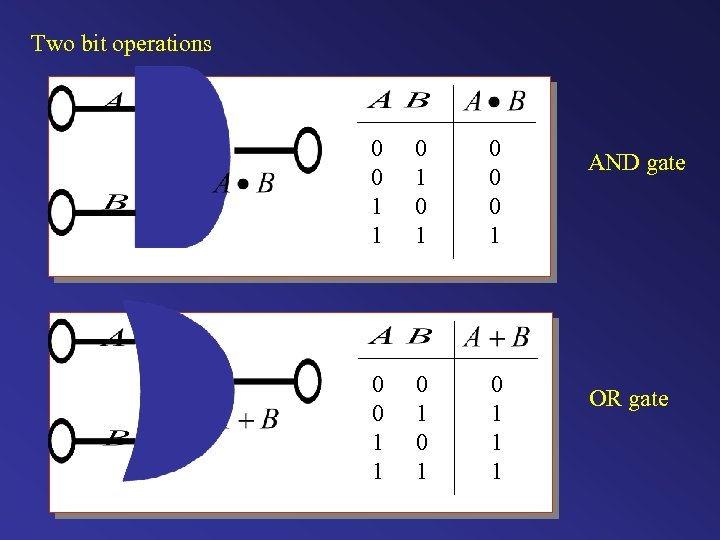Two bit operations 0 0 1 1 0 1 0 1 1 1 AND gate OR gate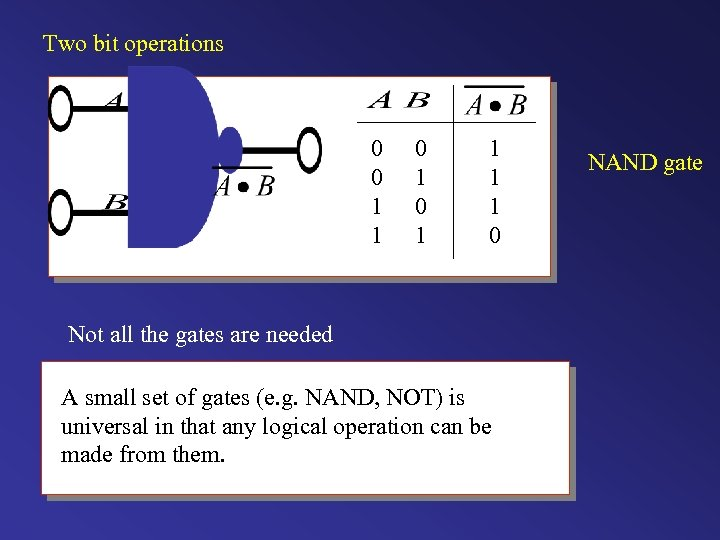Two bit operations 0 0 1 1 0 Not all the gates are needed A small set of gates (e. g. NAND, NOT) is universal in that any logical operation can be made from them. NAND gate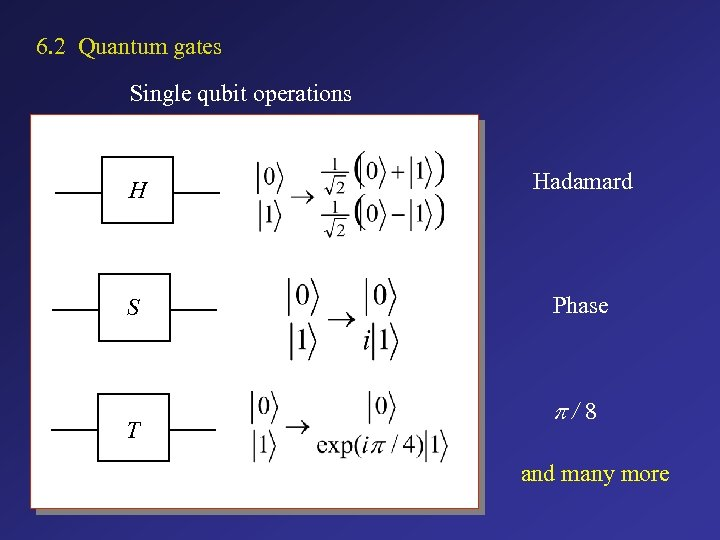6. 2 Quantum gates Single qubit operations H Hadamard S Phase T p/8 and many more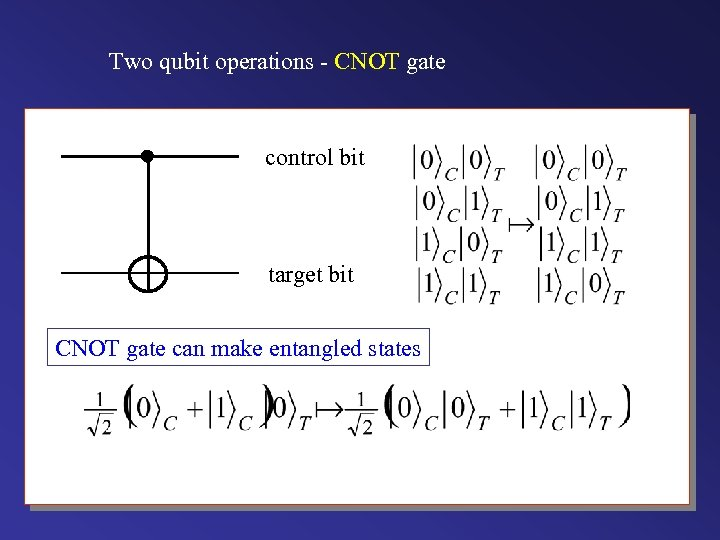Two qubit operations - CNOT gate control bit target bit CNOT gate can make entangled states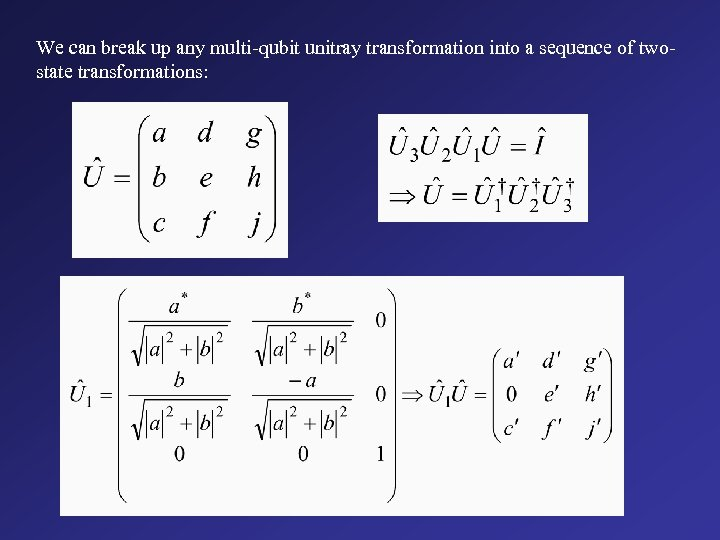We can break up any multi-qubit unitray transformation into a sequence of twostate transformations: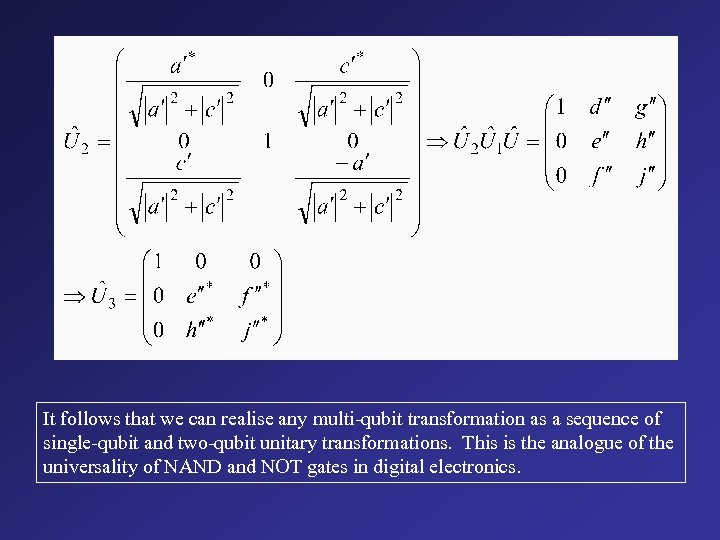It follows that we can realise any multi-qubit transformation as a sequence of single-qubit and two-qubit unitary transformations. This is the analogue of the universality of NAND and NOT gates in digital electronics.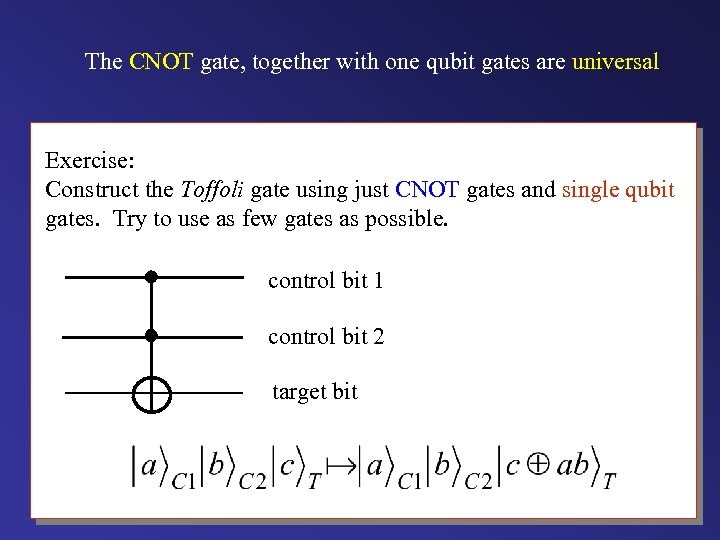The CNOT gate, together with one qubit gates are universal Exercise: Construct the Toffoli gate using just CNOT gates and single qubit gates. Try to use as few gates as possible. control bit 1 control bit 2 target bit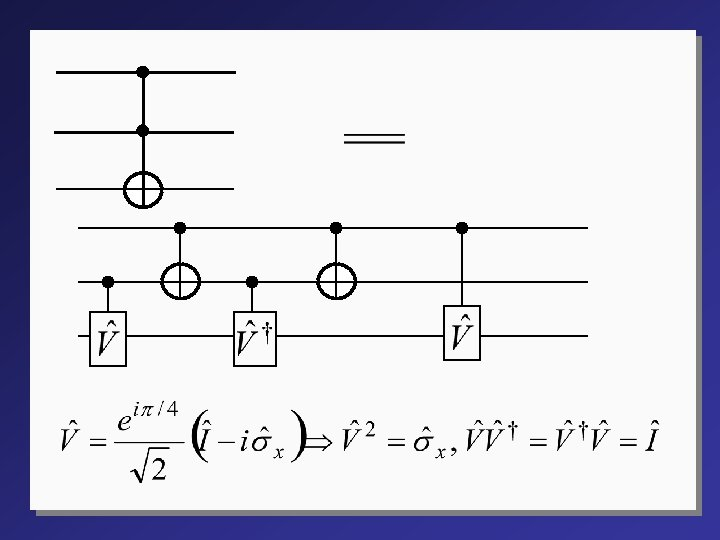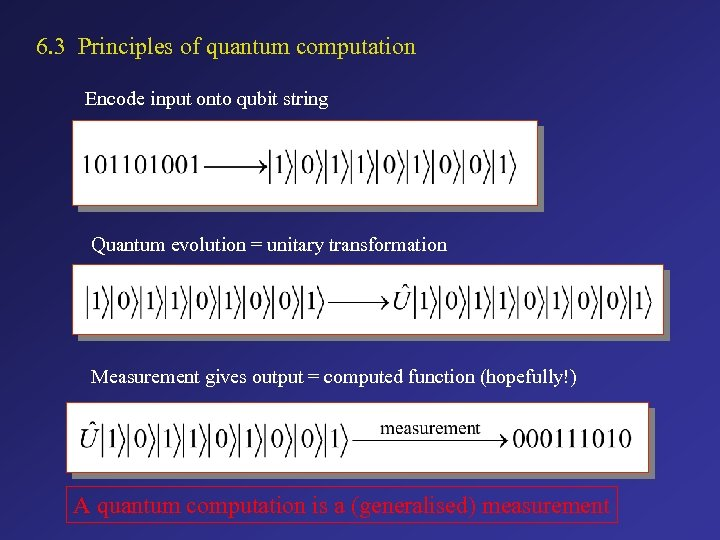6. 3 Principles of quantum computation Encode input onto qubit string Quantum evolution = unitary transformation Measurement gives output = computed function (hopefully!) A quantum computation is a (generalised) measurement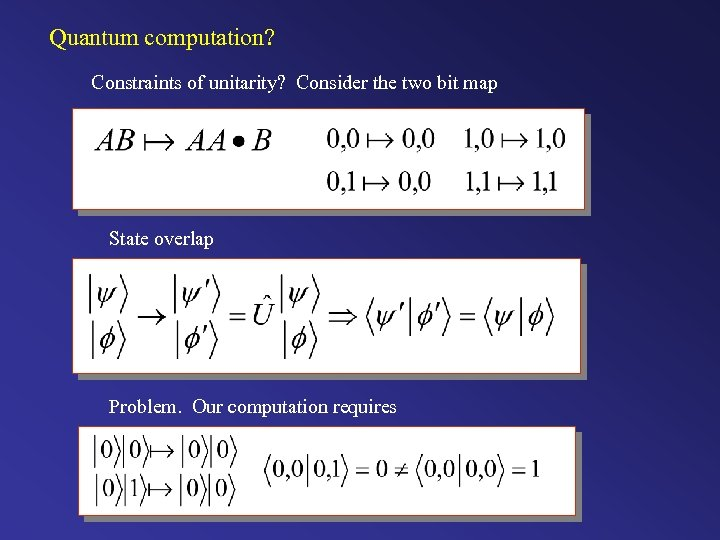Quantum computation? Constraints of unitarity? Consider the two bit map State overlap Problem. Our computation requires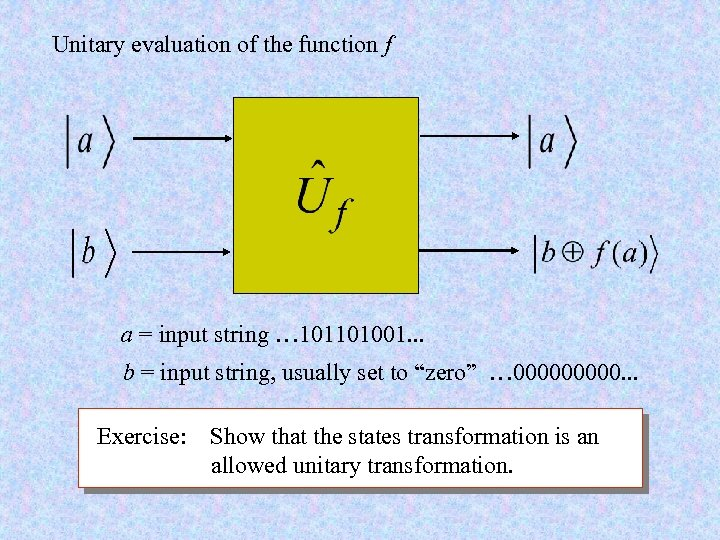Unitary evaluation of the function f a = input string … 101101001. . . b = input string, usually set to “zero” … 00000. . . Exercise: Show that the states transformation is an allowed unitary transformation.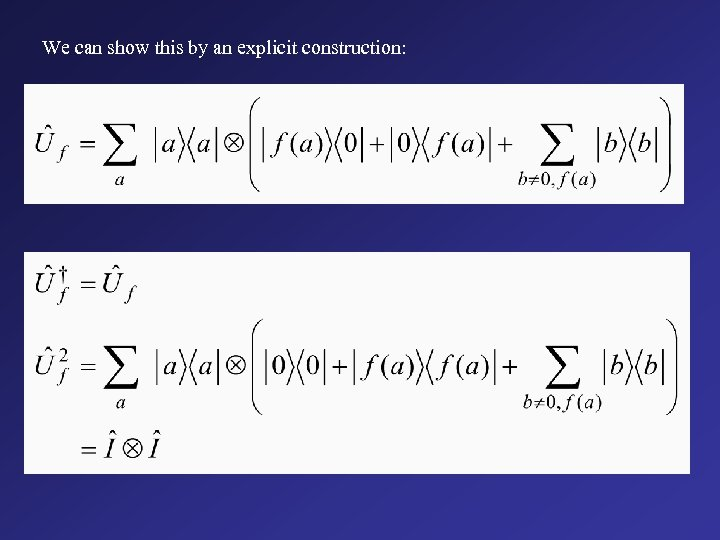We can show this by an explicit construction: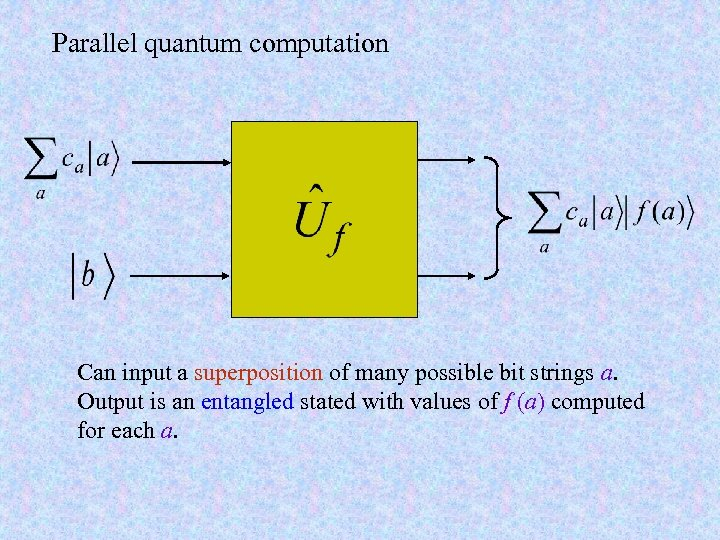Parallel quantum computation Can input a superposition of many possible bit strings a. Output is an entangled stated with values of f (a) computed for each a.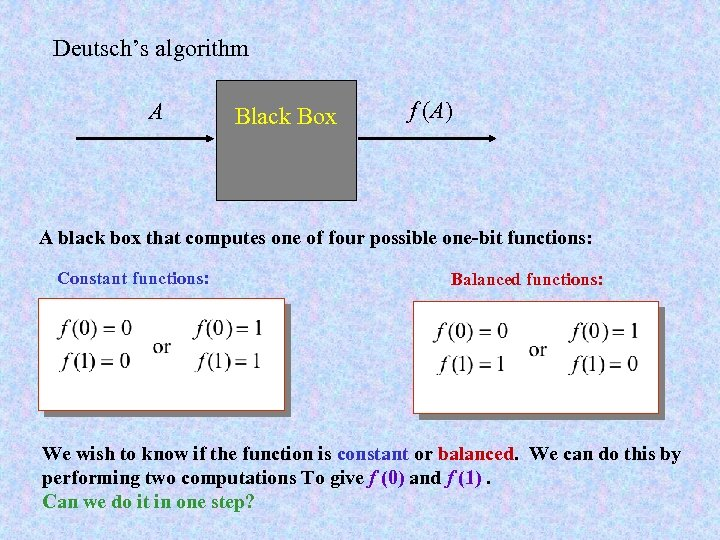Deutsch’s algorithm A Black Box f (A) A black box that computes one of four possible one-bit functions: Constant functions: Balanced functions: We wish to know if the function is constant or balanced. We can do this by performing two computations To give f (0) and f (1). Can we do it in one step?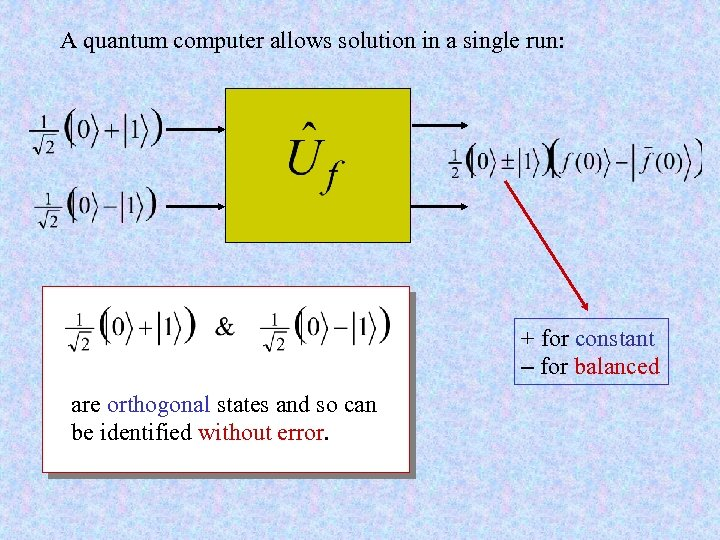A quantum computer allows solution in a single run: + for constant - for balanced are orthogonal states and so can be identified without error.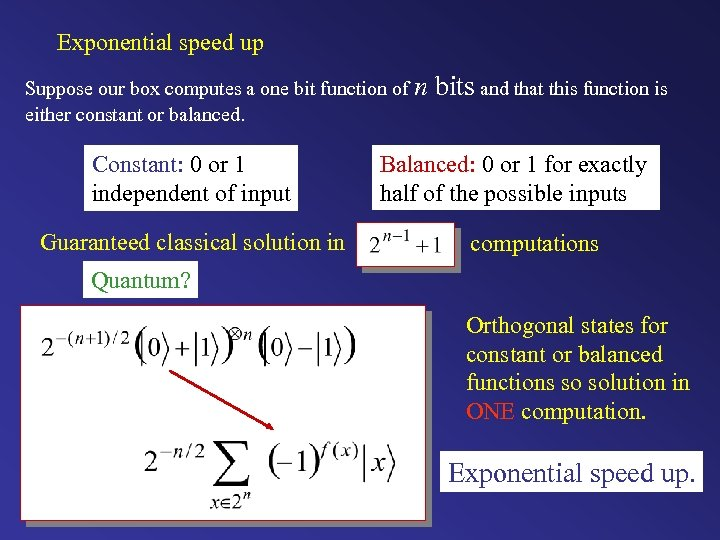Exponential speed up Suppose our box computes a one bit function of n either constant or balanced. Constant: 0 or 1 independent of input Guaranteed classical solution in bits and that this function is Balanced: 0 or 1 for exactly half of the possible inputs computations Quantum? Orthogonal states for constant or balanced functions so solution in ONE computation. Exponential speed up.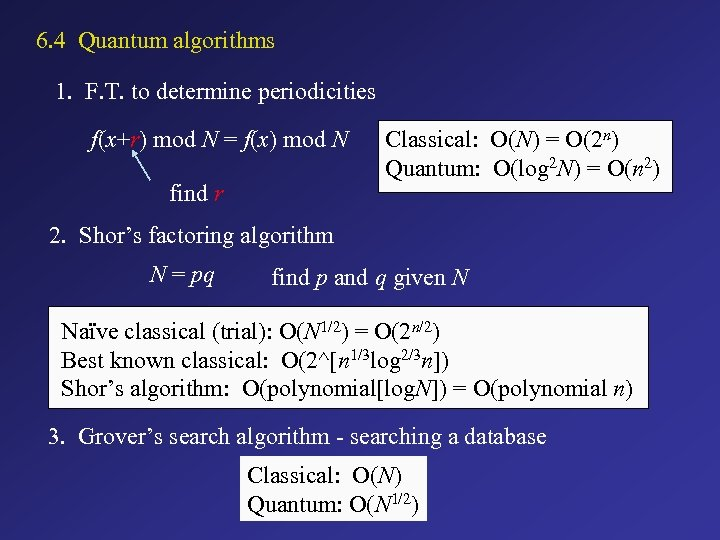6. 4 Quantum algorithms 1. F. T. to determine periodicities f(x+r) mod N = f(x) mod N find r Classical: O(N) = O(2 n) Quantum: O(log 2 N) = O(n 2) 2. Shor’s factoring algorithm N = pq find p and q given N Naïve classical (trial): O(N 1/2) = O(2 n/2) Best known classical: O(2^[n 1/3 log 2/3 n]) Shor’s algorithm: O(polynomial[log. N]) = O(polynomial n) 3. Grover’s search algorithm - searching a database Classical: O(N) Quantum: O(N 1/2)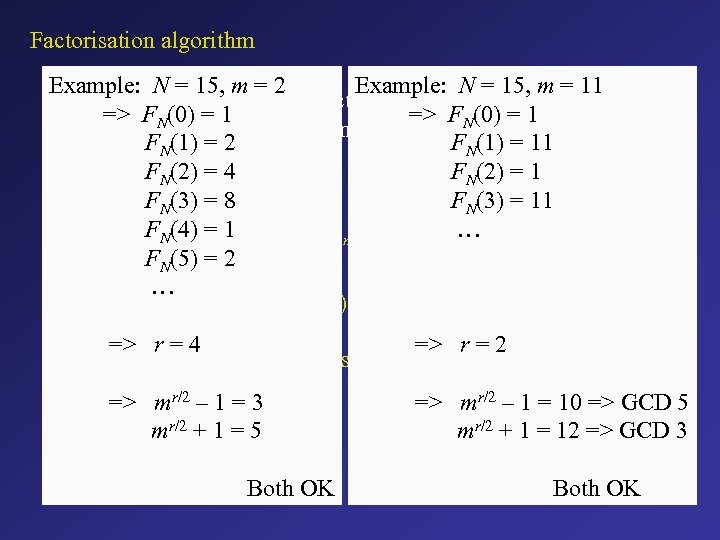Factorisation algorithm Example: N = 15, m = 2 Example: N = 15, m = 11 N: Given big integer to be factorised => FN(0) = 1 m: Small integer chosen at random FN(1) = 2 FN(1) = 11 n = 0, 1, 2, … FN(2) = 4 FN(2) = 1 FN(3) = 8 FN(3) = 11 FN(4) = 1 … n mod N 1. Make the series FN(n) = m FN(5) = 2 … 2. Find the period r : FN(n+r) = FN(n) => r = 4 => r = 2 3. The greatest common divisor of N and mr/2± 1 divides N => mr/2 – 1 = 3 mr/2 + 1 = 5 Both OK => mr/2 – 1 = 10 => GCD 5 mr/2 + 1 = 12 => GCD 3 Both OK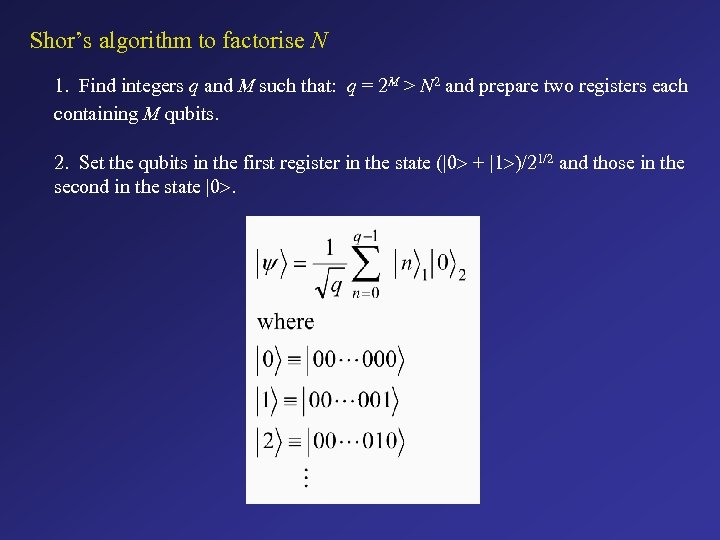Shor’s algorithm to factorise N 1. Find integers q and M such that: q = 2 M > N 2 and prepare two registers each containing M qubits. 2. Set the qubits in the first register in the state (|0> + |1>)/21/2 and those in the second in the state |0>.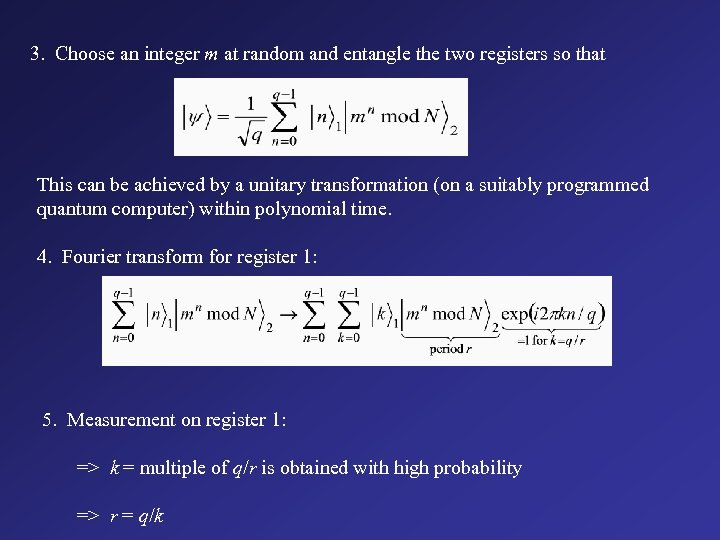3. Choose an integer m at random and entangle the two registers so that This can be achieved by a unitary transformation (on a suitably programmed quantum computer) within polynomial time. 4. Fourier transform for register 1: 5. Measurement on register 1: => k = multiple of q/r is obtained with high probability => r = q/k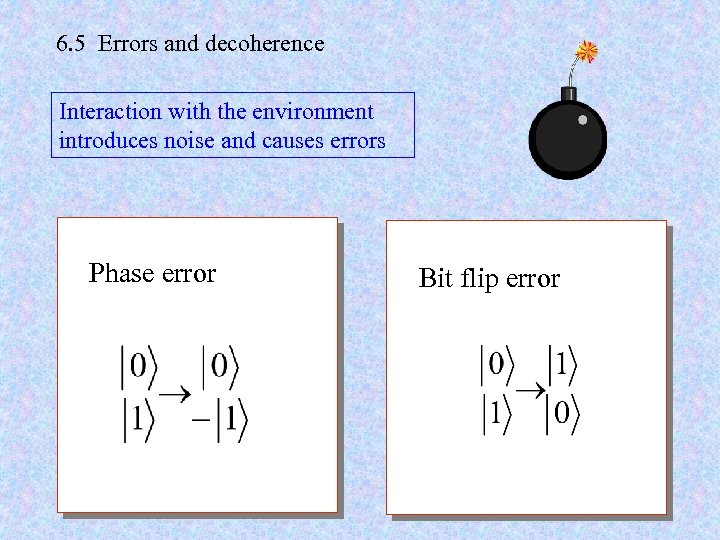6. 5 Errors and decoherence Interaction with the environment introduces noise and causes errors Phase error Bit flip error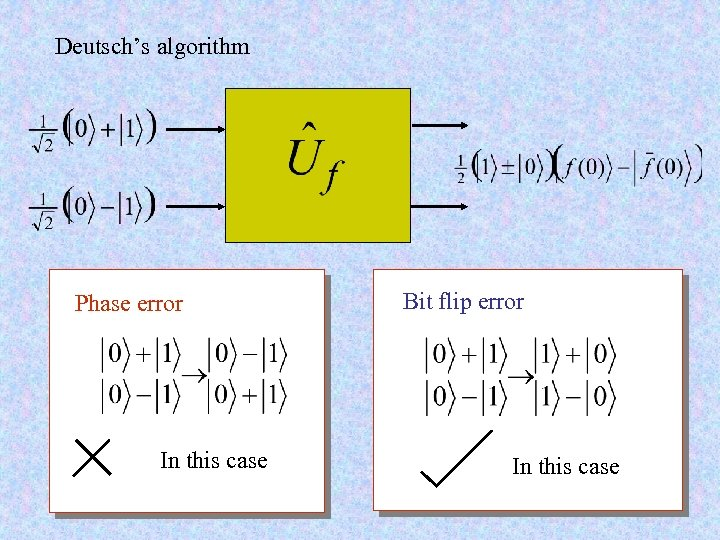Deutsch’s algorithm Phase error In this case Bit flip error In this case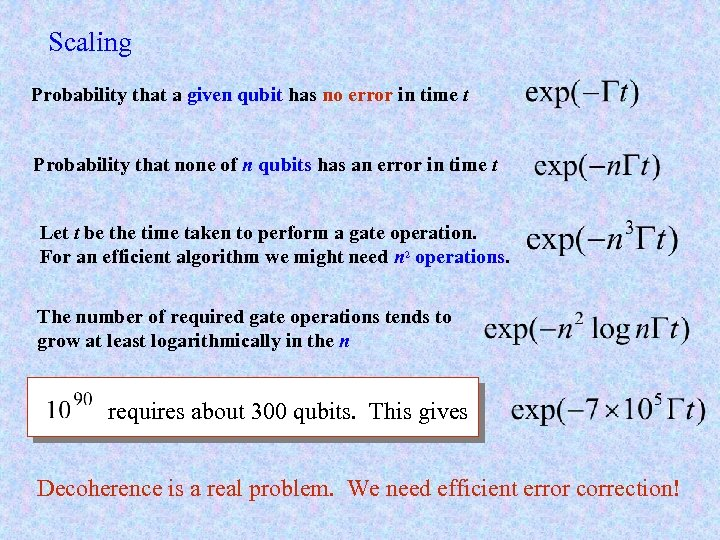Scaling Probability that a given qubit has no error in time t Probability that none of n qubits has an error in time t Let t be the time taken to perform a gate operation. For an efficient algorithm we might need n 2 operations. The number of required gate operations tends to grow at least logarithmically in the n requires about 300 qubits. This gives Decoherence is a real problem. We need efficient error correction!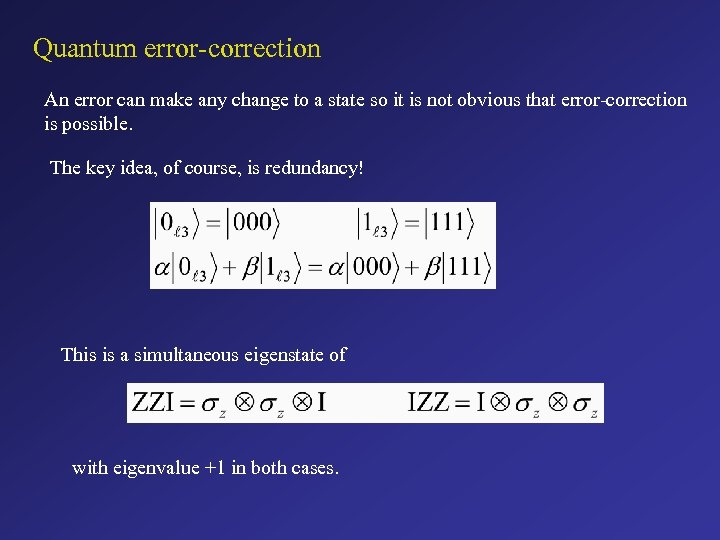Quantum error-correction An error can make any change to a state so it is not obvious that error-correction is possible. The key idea, of course, is redundancy! This is a simultaneous eigenstate of with eigenvalue +1 in both cases.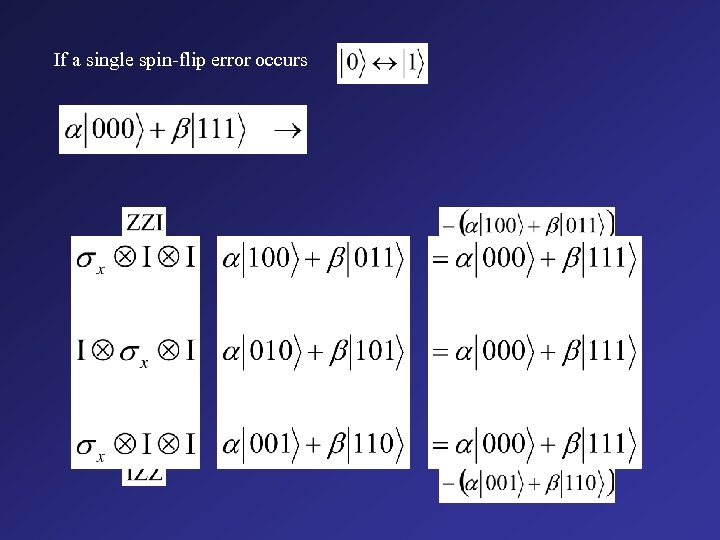If a single spin-flip error occurs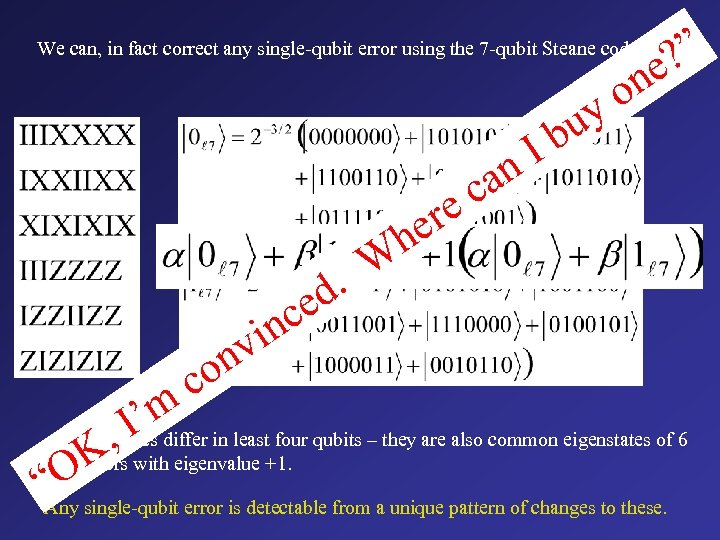We can, in fact correct any single-qubit error using the 7 -qubit Steane code: ? ” e on y an c e c W d. ce in nv o bu I er h ’m in least four qubits – they are also common eigenstates of 6 All the , I differ states K operators with eigenvalue +1. “Osingle-qubit error is detectable from a unique pattern of changes to these. Any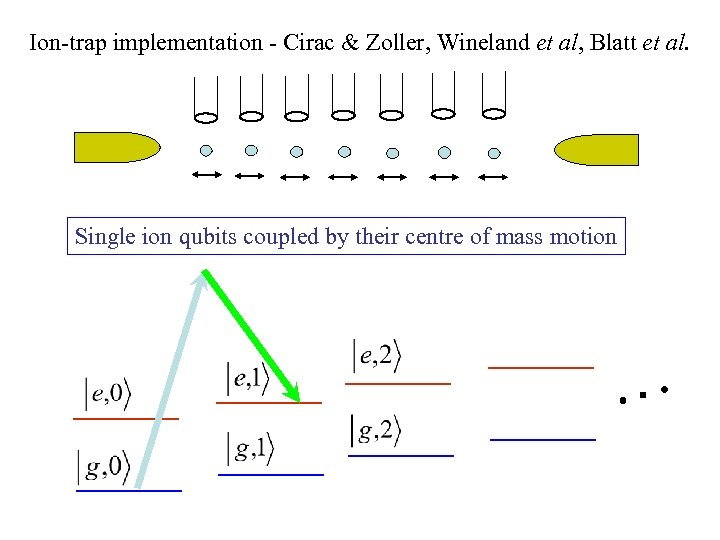Ion-trap implementation - Cirac & Zoller, Wineland et al, Blatt et al. Single ion qubits coupled by their centre of mass motion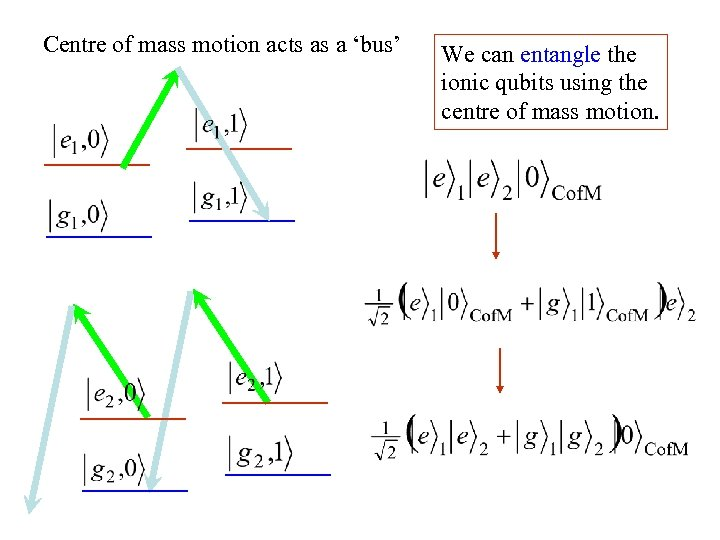Centre of mass motion acts as a ‘bus’ We can entangle the ionic qubits using the centre of mass motion.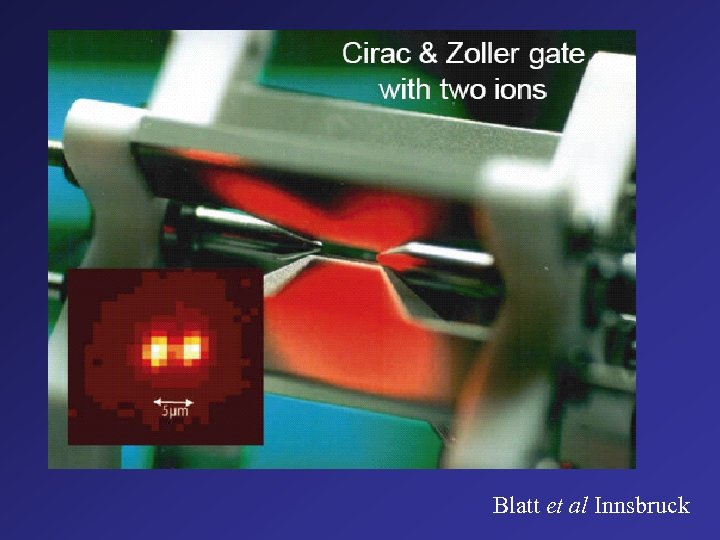Blatt et al Innsbruck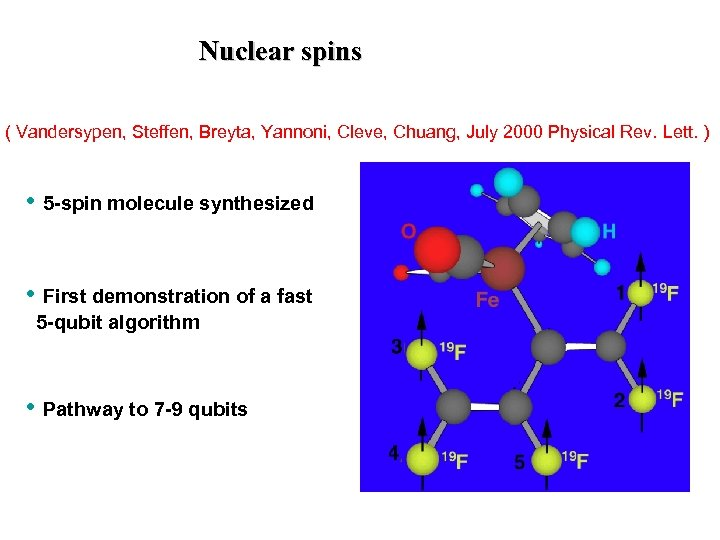Nuclear spins ( Vandersypen, Steffen, Breyta, Yannoni, Cleve, Chuang, July 2000 Physical Rev. Lett. ) • 5 -spin molecule synthesized • First demonstration of a fast 5 -qubit algorithm • Pathway to 7 -9 qubits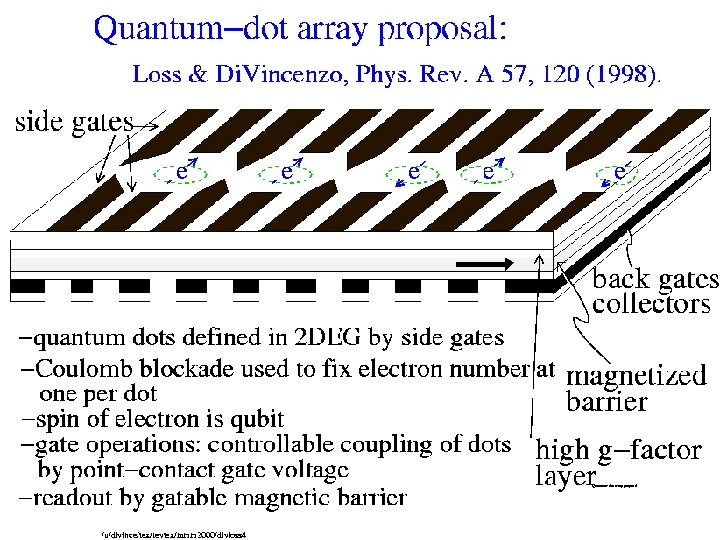Quantum-dot array proposal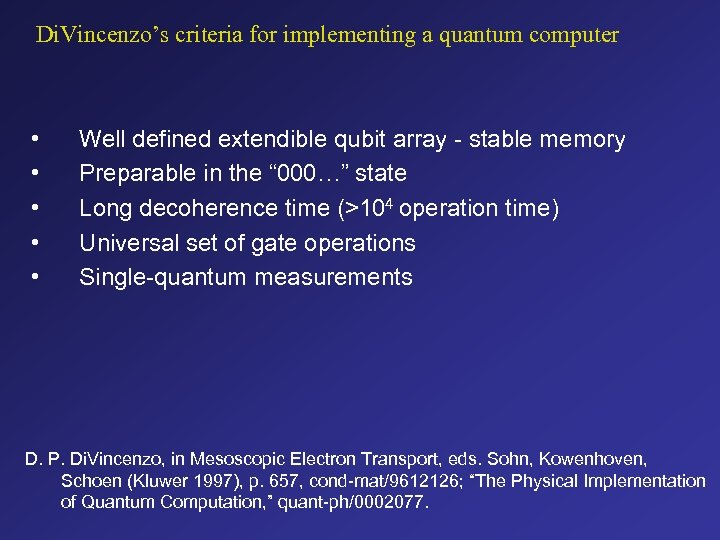Di. Vincenzo’s criteria for implementing a quantum computer • • • Well defined extendible qubit array - stable memory Preparable in the “ 000…” state Long decoherence time (>104 operation time) Universal set of gate operations Single-quantum measurements D. P. Di. Vincenzo, in Mesoscopic Electron Transport, eds. Sohn, Kowenhoven, Schoen (Kluwer 1997), p. 657, cond-mat/9612126; “The Physical Implementation of Quantum Computation, ” quant-ph/0002077.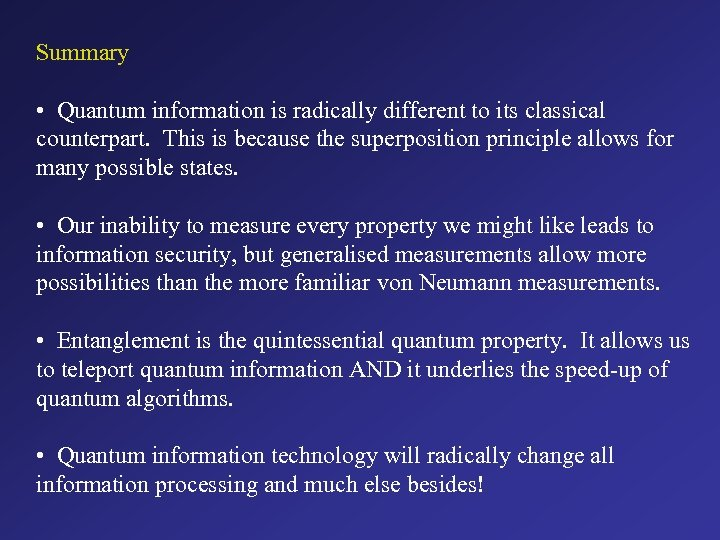Summary • Quantum information is radically different to its classical counterpart. This is because the superposition principle allows for many possible states. • Our inability to measure every property we might like leads to information security, but generalised measurements allow more possibilities than the more familiar von Neumann measurements. • Entanglement is the quintessential quantum property. It allows us to teleport quantum information AND it underlies the speed-up of quantum algorithms. • Quantum information technology will radically change all information processing and much else besides!